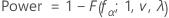# Methods and formulas for Power and Sample Size for 2-Level Factorial Design

Select the method or formula of your choice.

## Calculating power

### Calculating power

Minitab assumes that there are no text factors, and therefore no pseudo center points.

Minitab first calculates the degrees of freedom for error (v).

Degrees of freedom for error
ν = (total number of observations – number of parameters estimated)
total number of observations = n * r + cptotal
number of parameters estimated = number of unique corner points per replicate + 1 (if term for center points is included) + number of parameters for blocks (if blocks are in the model) – number of terms omitted from model – number of factorial terms confounded with blocks
The number of parameters for blocks is b-1. If n = b or if n/b is an integer, then no factorial terms are confounded with blocks.
If n/b is not an integer, each block has only a fraction 1/2q of the base design. Let q be the smallest integer for which n is a multiple of b/2q. Then 2q – 1 factorial terms are confounded with blocks.
Power### Notation

TermDescription
k number of factors
r number of runs at the corner points per replicate
n number of replicates
b number of blocks (if no blocking exists, b = 1))
σ estimated standard deviation
δ effect
α significance level
v degrees of freedom for error
λ noncentrality parameter
fα critical value (upper α point of the F distribution with 1 and ν degrees of freedom)
F(fα ; 1, v, λ)CDF of the F distribution with 1 numerator degree of freedom, v denominator degrees of freedom, and noncentrality parameter λ, evaluated at fα
cpblocknumber of center points per block
cptotaltotal number of center points = b * cpblock

## Calculating replicates, effects, and center points

If you provide values for power and two other properties, Minitab calculates the unknown fourth property by applying the power equation iteratively. At each iteration, Minitab evaluates the power for the values of the properties that you provide and a trial value of the statistic that you did not provide. Minitab stops when the algorithm reaches the power value that you specified.

When you provide a power value, Minitab may find that no integer number of replicates yields your target power. In such cases, Minitab displays the target value for power alongside the actual power attainable given your specifications. The actual power is a value nearest to, yet greater than, the target power.

## Noncentrality parameter

### Notation

TermDescription
r number of runs at the corner points per replicate
n number of replicates
σ estimated standard deviation
δ effect
λ noncentrality parameter
By using this site you agree to the use of cookies for analytics and personalized content.  Read our policy